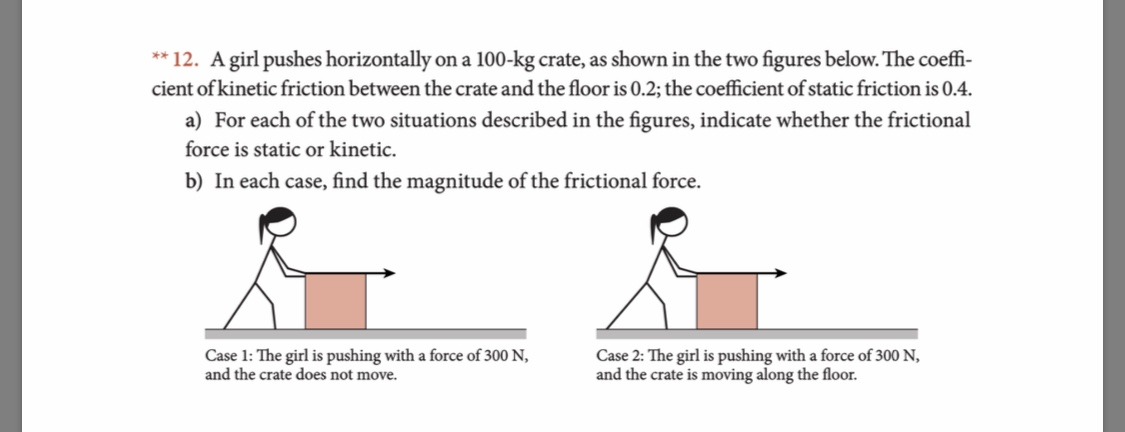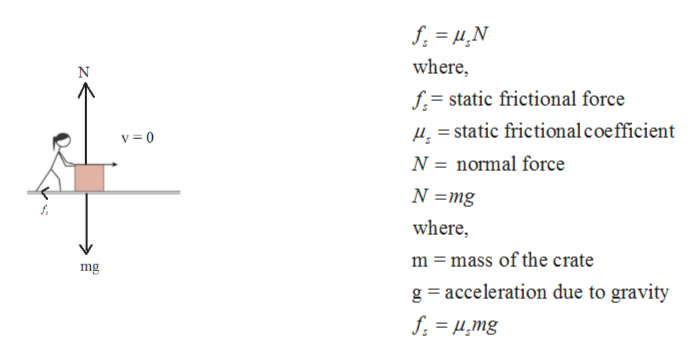# ** 12. A girl pushes horizontallycient of kinetic friction between the crate and the floor is 0.2; the coefficient of static friction is 0.4.on a 100-kg crate, as shown in the two figures below. The coeffi-a) For each of the two situations described in the figures, indicate whether the frictionalforce is static or kinetic.b) In each case, find the magnitude of the frictional force.Case 1: The girl is pushing with a force of 300 N,and the crate does not move.Case 2: The girl is pushing with a force of 300 N,and the crate is moving along the floor.

Question
135 viewshelp_outlineImage Transcriptionclose** 12. A girl pushes horizontally cient of kinetic friction between the crate and the floor is 0.2; the coefficient of static friction is 0.4. on a 100-kg crate, as shown in the two figures below. The coeffi- a) For each of the two situations described in the figures, indicate whether the frictional force is static or kinetic. b) In each case, find the magnitude of the frictional force. Case 1: The girl is pushing with a force of 300 N, and the crate does not move. Case 2: The girl is pushing with a force of 300 N, and the crate is moving along the floor. fullscreen
check_circle

Step 1

Given:

Mass of the crate = 100 kg

Coefficient of static friction µs = 0.4

Coefficient of kinetic friction µk = 0.2

Step 2

Case 1st :

The girl is pushing but the crate does not move therefore frictional force is static.

Case 2nd :

In this case the crate is moving therefore frictional force is kinetic.

Step 3

Case 1st :

Magnitude of static friction...help_outlineImage Transcriptionclosewhere f=static frictional force static frictional coe fficient N normal force v0 N=mg where, m mass of the crate mg g acceleration due to gravity f = ,mg fullscreen

### Want to see the full answer?

See Solution

#### Want to see this answer and more?

Solutions are written by subject experts who are available 24/7. Questions are typically answered within 1 hour.*

See Solution
*Response times may vary by subject and question.
Tagged in

### Friction